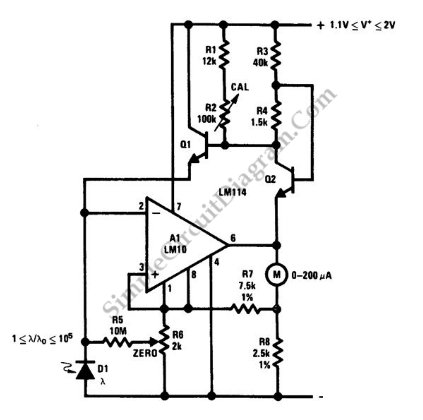# Five-Decade Dynamic Light-Level Meter

This is a Portable light-level meter circuit with five-decade dynamic range. This uses  a single cell battery as the power supply. To calibrate this circuit, use an appropriate illumination, then adjust R2 so that the output equals the reference. To make this circuit give output meter follows standard light meter, adjust R2 and R6. Maybe there is an error at the scale extremes because the log slope is not temperature compensated. Here is the schematic diagram of the circuit:An infrared-stop filter is recommended for this circuit because silicon photo-diodes (D1) are sensitive to near-infrared light. This circuit will give a excellent results if an appropriate correction filter  is applied and a blue-enhanced photodiode for D1 is used. [Circuit’s schematic diagram source: National Semiconductor Application Note]